# NCERT Solutions for Class 6 Maths Chapter 10 Mensuration

NCERT Solutions for Class 6 Maths Chapter 10 Mensuration: It is a process of measurement which is needed for different geometric shapes both 2D and 3D shapes. For 2-D shapes, you can measure the area (A) and perimeter(P). The distance covered along the boundary of a closed figure is known as perimeter and the space occupied by a closed figure is known as area. In CBSE NCERT solutions for class 6 maths chapter 10 mensuration, you will get many questions related to calculating the perimeter and area of a rectangle, square and a triangle. For 3-D shape, you can calculate total surface area, lateral surface area, curved surface area and volume (V). This chapter is more focused on the measurement of 2-D shapes. In class 8 you will learn measurement for 3-D shapes also. If you have given a complex shape to calculate its area, you can divide it into smaller parts and the sum of all these parts will be equal to the total area of that shape. In solutions of NCERT for class 6 maths chapter 10 mensuration, there are some problems where you have to find areas of figures by counting the number of squares and sum of their area is the total area of that figure. When you go through these problems it will give you more clarity.  There are 30 questions given in the 3 exercises of this chapter. All these questions are explained in CBSE NCERT solutions for class 6 maths chapter 10 mensuration. It will help you to understand the concept in a more easy way. Check NCERT solutions from class 6 to 12 to learn science and maths

Topic 10.2

Topic 10.2.1

Topic 10.3.1

Exercise 10.1

Exercise 10.2

Exercise 10.3

## The main topics of the Solutions for NCERT Class 6 Maths Chapter 10 Mensuration are:

10.1 Introduction

10.2 Perimeter

10.2.1 Perimeter of a rectangle

10.2.2 Perimeter of regular shapes

10.3 Area

10.3.1 Area of a rectangle

10.3.2 Area of a square

All NCERT Solutions of the chapter are mandatory and we need to practice all the questions.

## Question:3 Meera went to a park 150 m long and 80 m wide. She took one complete round on its boundary. What is the distance covered by her?

The park is 150m long and 80 m wide. The parks are of the shape of the rectangle. The distance covered by Meera = perimeter of park = 2 (150+80)= 460 m

Question:4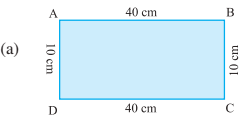Perimeter = AB + BC + CD + DA
= __+__+__+__
= ______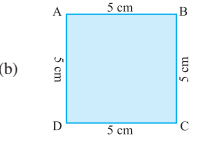Perimeter = AB + BC + CD + DA
= __ + __ + __+ __
= ______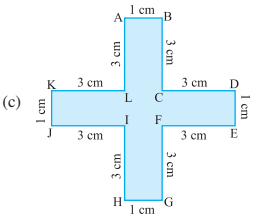Perimeter = AB + BC + CD + DE + EF + FG + GH +HI + IJ + JK + KL + LA
= __ + __ +__ + __ + __ +  __ + __ + __ +__+ __ + __ + __
= ______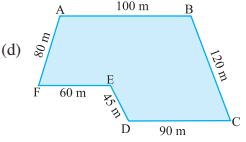Perimeter = AB + BC + CD + DE + EF+ FA
= __ + __ + __ + __ + __ + __
= ______

Find the perimeter of the following figures:Perimeter = AB + BC + CD + DA
= 40+10+40+10
= 100Perimeter = AB + BC + CD + DA
=5+5+5+5=20Perimeter = AB + BC + CD + DE + EF + FG + GH +HI + IJ + JK + KL + LA
=1+3+3+1+3+3+1+3+3+1+3+3=28 cmPerimeter = AB + BC + CD + DE + EF+ FA
=100 + 120 + 90 + 45+ 60 +80=495 cm

CBSE NCERT for class 7 maths chapter 10 mensuration topic 10.2.1 perimeter of a rectangle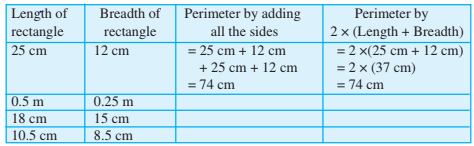Length of rectangle Breadth of rectangle Perimeter by adding all sides perimeter by 25 cm 12 cm =25+12+25+12=74 cm 0.5 cm 0.25cm =0.5+0.25+0.5+0.25=1.5cm 18 cm 15 cm =18+15+18+15=66 cm 10.5 cm 8.5 cm =10.5+8.5+10.5+8.5=38 cm

NCERT solutions for class 6 maths chapter 10 mensuration-Exercise: 10.1The perimeter of the following figures-
Perimeter = sum of all sides

Therefore,
(a) Perimeter = 4 cm + 2cm +1 cm +5cm = 12 cm

(b) Perimeter = 23 cm + 35 cm + 40cm + 35cm = 133 cm

(c) Required perimeter = 15cm + 15 cm + 15 cm +15 cm = 60 cm

(d) Required perimeter= (4 + 4 + 4 + 4 + 4) cm = 20 cm

(e) Perimeter = 4cm + 0.5 cm +2.5cm +2.5cm + 0.5cm +4cm +1cm = 15cm

(f) Perimeter = Sum of all sides = 4(4cm) + 4(1 cm) + 8(3 cm) + 4(2cm) = (16 + 4 + 24 + 8)cm =52 cm

We have,
Length = 40 cm and,

So, the length of tape required = perimeter of the rectangular box
= 2 (L + B)
= 2 50 cm
= 100 cm

Hence 100 cm tape is required.

We have table-top measures 2 m 25 cm by 1 m 50 cm.
Therefore, the Perimeter of the table-top = sum of all sides
= 2(L+B)
= 2 ((2m + 0.25m) + (1m + 0.5m))
= 7.5 m

We have,
Length and breadth of a photograph is 32 cm and 21 cm respectively.

So, According to question,
Length of wooden strip required to frame the photograph = 2 [L + B]
= 2 [32 + 21] cm
= 2   53 cm  = 106 cm

Hence the required length of the strip is 106 cm or 1.06 m

We have,
A rectangular piece of land measures 0.7 km by 0.5 km and side is to be fenced with 4 rows of wires.

As per the question,

Required length of wires
= 4 2 [ 0.7 km + 0.5 km]
= 8  1.2 km
= 9.6 km

(a) A triangle of sides 3 cm, 4 cm and 5 cm.

(b) An equilateral triangle of side 9 cm.

(c) An isosceles triangle with equal sides 8 cm each and third side 6 cm.

(a) The perimeter of a triangle = Sum of all the three sides
= 3cm + 4cm + 5cm
= 12 cm

(b) The perimeter of a eqilateral triangle
= Sum of all the sides of  the triangle
= (9cm +9cm +9cm)
= 27cm

(c) Perimeter of a given isosceles triangle
= Sum of all sides
= 8cm + 8cm +6cm
= 22 cm

We all know that,
Perimeter of a triangle = sum of all the sides of the triangle
=10cm + 14cm +15cm
= 39 cm

It is known that,
A regular hexagon has six equal sides.
So, the perimeter of the regular hexagon
= 6  side
= 6  8m = 48m

Given that,
The perimeter of the square is 20m
According to question,
The perimeter of square = 4  side

Given,
The perimeter of a regular pentagon is 100cm and,
no. of sides in regular pentagon is five (5)
therefore, the length of each side

(a) a square?             (b) an equilateral triangle?             (c) a regular hexagon?

Given that,

Length of the string is 30 cm
(a)For square,
Number of sides = 4
Therefore, Length of one side = 30 /4 = 7.5 cm

(b)For equilateral triangle,
Number of sides = 3
Therefore, length of sides = 30/3 = 10cm

(c) For a regular hexagon,
Number of sides = 6
Therefore, Length of one side = 30/6 = 5cm

Given that,
The two sides of the triangle are 12 cm and 14 cm. let the third side be A.
And the perimeter of the triangle is 36cm.

Therefore, the Perimeter of the triangle
= sum of all sides of the triangle
= 12cm +14cm + A = 36cm
= A + 26cm =36cm
= A = 10cm

Given that,
Length of the square park  = 250 m

Cost of fencing = rs. 20 per meter

Therefore, the Perimeter of the square park =
= 4  250
=1000 m
= 1km

So, total cost = 20   1000 = 20,000 Rs

Given that,
The length of the rectangular park is 15 m and the breadth is 125m.
Cost of fencing rate = Rs 12 per meter

Therefore,
Perimeter of the park
=2 [L + B]
= 2 [175 +125]
=2  300m
=600m

So, the total cost of fencing = 600   12  = Rs. 7,200

Given that,
Length of the square park = 75m
Length and breadth of the rectangular park is 60 m and 45m respectively

Therefore,
The perimeter of the square park
= 4  75
=300 m

Also, the Perimeter of the rectangular park
= 2 ( 60 + 45)
=2 105m
=210m

Hence Bulbul covers the less distance.(a) The perimeter of the square
= Sum of all the four side = 4   side
= 4   25
= 100 cm

(b)The perimeter of the rectangle
= SUm of all the sides = 2  [length + breadth )
= 2  (20 + 30)
= 2  50
= 100

(c)The perimeter of the rectangle
= Sum of all the sides = 2  [length + breadth ]
= 2  (40 + 10)
= 2  50
= 100 cm

(d)The perimeter of the triangle
= Sum of all three sides of the triangle
= 30cm + 30cm + 40cm
=100cm

We conclude all the given figures have the same perimeter.

(a) What is the perimeter of his arrangement ?

(b) Shari does not like his arrangement. She gets him to lay them out like a cross. What is the perimeter of her arrangement?

(c) Which has greater perimeter?

(d) Avneet wonders if there is a way of getting an even greater perimeter. Can you find a way of doing this?Given that,
Length of each slab =
So, the length of the square =

(a) The first figure is a square
So, the perimeter of the square = 4  side
=

(b) The perimeter of the 2nd figure
= Sum of all the sides

= 10 m

(c) Figure (ii) has a greater perimeter than fig (i)

(d) Arrange all the slab in horizontally, it forms a rectangle whose dimensions are

## NCERT Solutions for class 6 maths chapter 10 mensuration-Exercise: 10.2Area of 1 square = 1sq. unit

(a) Number of total squares = 9
Therefore, the area of 9 squares = 9 sq. units

(b) In fig (b) the total number of square =  5
Therefore, are = 5 sq units

(c) In fig (c) the number of squares = 4
So, the area is 4 sq. units

(d) In fig (d) number of squares = 8
Therefore, area = 8 sq. units

(e) Total number of square = 10
therefore, area = 10 sq. units

(f) total number of squares = 4
Therefore, area = 4 sq. units

(g)  Total number of square = 6
therefore, area = 6 sq. units

(h) Total number of square = 5
therefore, area = 5 sq. units

(i)  Total number of square = 9
therefore, area = 9 sq. units

(j)  Total number of square = 4
therefore, are = 4 sq. units

(k)  Total number of square = 5
therefore, area = 5 sq. units

(l) Total number of full squares = 4
Squares more than half = 3
half squares = 2
Therefore, the total area  = 8 sq. units

(m) Count more than half squares as 1
Now, the total number of squares = 14
Therefore, the total area = 14 sq. unit

(n) Count more than half squares as 1
Now, the total number of squares = 18
Therefore, the total area = 18 sq. unit

## Solutions for NCERT class 6 maths chapter 10 mensuration topic 10.3.1 area of a rectangle

If floor length is 3m and breadth is 2m then

suppose the length of door is 2m and breadth is 0.5 m

then the area of door =

CBSE NCERT solutions for class 6 maths chapter 10 mensuration-Exercise: 10.3

(a) 3 cm and 4 cm
(b) 12 m and 21 m
(c) 2 km and 3 km
(d) 2 m and 70 cm

We know that area of a rectangle = length  breadth
Therefore,
(a) Here length is 3cm and the breadth of the rectangle is 4cm
So, the area of the rectangle
=
(b) Here length is 12m and the breadth of the rectangle is 21m
So, the area of the rectangle
=
(c) Here length is 3km and the breadth of the rectangle is 3km
So, the area of the rectangle ?
=
(d)  Here length is 2m and the breadth of the rectangle is 70cm =
So, the area of the rectangle
=

(a) 10 cm                 (b) 14 cm             (c) 5 m

It is known that area of a square =

(a) The length of a side of a square = 10cm
So, the area =

(b)The length of a side of a square = 14cm
So, the area =

(c) The length of a side of a square = 5m
So, the area =

(a) 9 m and 6 m                 (b) 17 m and 3 m                 (c) 4 m and 14 m

Which one has the largest area and which one has the smallest?

We know that area of a rectangle = length  breadth
Therefore,
(a) Here length is 9m and the breadth of the rectangle is 6m
So, the area of the rectangle
=

(b) Here length is 17 m and the breadth of the rectangle is 3m
So, the area of the rectangle
=

(c) Here length is 4m and the breadth of the rectangle is 14m
So, the area of the rectangle
=

Hence rectangle C has the greatest area and rectangle B has the least area.

Given that,
Area of the rectangular garden = 300 sq. m
and the length = 50 m

Therefore, the Width of the garden =
=

Hence the width of the garden is 6 m

Given that,
Length of the rectangular plot = 500m
and the width = 200 m
fencing cost  = Rs 8 per hundred sq. meter

Therefore, the area of the plot
=

Now, Total rate of tiling of 100,1000 m2   =
= Rs 8000

Given that,
Length of the table-top = 2m
and it's breadth = 1.5 m

Therefore, the area of the tabletop

Given that,
Length of the room = 4 m
And it's breadth = 3.5 m
Area of the room = = length  breadth

=

Hence the area of carpet needed is

Given that,
Length of the floor = 5 m
and its width = 4 m
Therefore,  the area of the floor

And, side of the square carpet = 3m
Therefore,  the area of the square carpet =
= 3m  3m = 9m2

Thus, the area of the floor which is not covered with carpet =

Given that,
Length and breadth of the land are 5m and 4 m.
Side of the square flower bed = 1m
Therefore the area of the bed = 1

So, Area of 5 square flower bed = 5

Area of the and = length  breadth
=

Thus, the area of the remaining part of the land = Area of land - area of the flower bed
= 20  - 5  = 15(a) Split the given figures into small rectangular parts as shown here;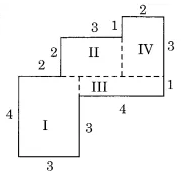Now, the area of the rectangle (I) =  = 4  3 = 12
the area of the rectangle (II) =  = 3  2= 6
the area of the rectangle (III) =  = 4  1 = 4
the area of the rectangle (IV) =  = 3  2 = 6

So, the total area of the figure = sum area of all rectangles
= (12 + 6 + 4 + 6)
= 28

(b) Split the given figure into smalle rectangles  as shown here;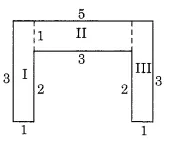the area of the rectangle (I) =  = 3  1 = 3
the area of the rectangle (II) =  = 3  1= 3
the area of the rectangle (III) =  = 3  1 = 3 ?

Therefore, the total area of the figure = sum of the area of all the small rectangles
=
=(a) Split the given figure into a small rectangle, as shown here;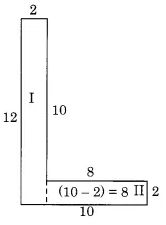Now, the area of the rectangle (I) =  = 12  2 =
the area of the rectangle (II) =  = 8  2=

Therefore, Total area =

(b) Split the given figure into a small rectangle, as shown here;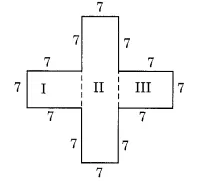Now, the area of the rectangle (I) =  = 7 7 =
the area of the rectangle (II) =  = 21  7=
Now, the area of the rectangle (III) =  = 7 7 =

Therefore, Total area =

(c) Split the given figure into a small rectangle, as shown here;Now, the area of the rectangle (I) =  = 2  1 =
the area of the rectangle (II) =  = 5  1=
Now, the area of the rectangle (III) =  = 2 1 =

Therefore, Total area =

(a) 100 cm and 144 cm
(b) 70 cm and 36 cm.

Given that,
the length and breadth of the tiles are 12 cm and 5 cm

Therefore, the area of the tiles
= 12 cm  5 cm
= 60 cm2

Now, (a) For 100 cm and 144 cm rectangular region,
Area of region =  length  breadth
= 14,400 cm2

Therefore, the number of tiles needed =  tiles

(b)  For 70 cm and 36 cm rectangular region,
Area of region =  length  breadth
= 70 cm  36 cm
= 2520 m2

Required number of tiles =

NCERT Solutions for Class 6 Mathematics - Chapter-wise

 Chapters No. Chapters Name Chapter - 1 Solutions of NCERT for class 6 maths chapter 1 Knowing Our Numbers Chapter - 2 CBSE NCERT solutions for class 6 maths chapter 2 Whole Numbers Chapter - 3 NCERT solutions for class 6 maths chapter 3 Playing with Numbers Chapter - 4 Solutions of NCERT for class 6 maths chapter 4 Basic Geometrical Ideas Chapter - 5 CBSE NCERT solutions for class 6 maths chapter 5 Understanding Elementary Shapes Chapter - 6 NCERT solutions for class 6 maths chapter 6 Integers Chapter - 7 Solutions of NCERT for class 6 maths chapter 7 Fractions Chapter - 8 NCERT solutions for class 6 maths chapter 8 Decimals Chapter - 9 CBSE NCERT solutions for class 6 maths chapter 9 Data Handling Chapter -10 NCERT solutions for class 6 maths chapter 10 Mensuration Chapter -11 Solutions of NCERT for class 6 maths chapter 11 Algebra Chapter -12 CBSE NCERT solutions for class 6 maths chapter 12 Ratio and Proportion Chapter -13 NCERT solutions for class 6 maths chapter 13 Symmetry Chapter -14 Solutions of NCERT for class 6 maths chapter 14 Practical Geometry

## NCERT Solutions for Class 6 - Subject wise

Some important formulas from the NCERT solutions for class 6 maths chapter 10 mensuration-

• Perimeter of rectangle:

Perimeter = 2 × (length + breadth)Area of the rectangle with length l and breadth b is the product of length and breadth = lb. The perimeter= l+b+l+b=2(l+b)

• Perimeter of a square:

Perimeter= 4 × length of its side

• The perimeter of a triangle:

Perimeter = (a+b+c)

a- First side of a triangle

b- Second side of a triangle

c- Third side of a triangle

For an equilateral triangle, all the sides are equal so its perimeter is 3l.

l- side of an equilateral triangle

• Area of a rectangle:

Area = length(l) × breadth (b)

• Area of a square:

Area = (side x side) A triangle has three sides.

You can derive all these formulas from the basic concepts of this chapter. There many questions in CBSE NCERT solutions for class 6 maths chapter 10 mensuration where you will find the complex geometry, to solve that you have to use your basic concepts of the geometry.